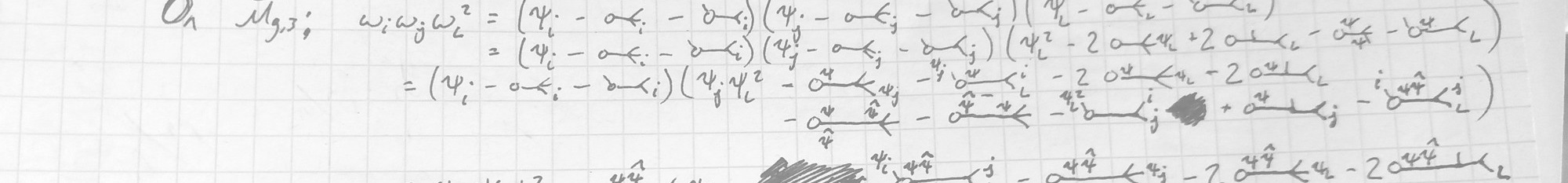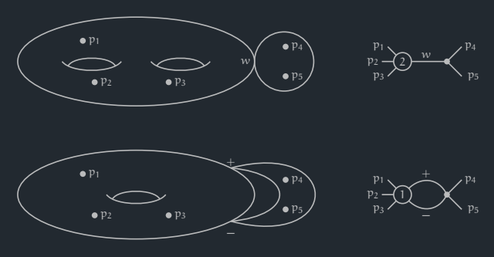# Home Research Teaching LifeMy research interests are primarily in studying moduli spaces of curves and their Chow rings. In particular I have focused on using the combinatorial description of these rings' intersection products to study families of effective classes. I am also interested in related material, such as more general moduli space theory, Gromov-Witten theory, and CohFTs.

My current specific topics of interest include the geometric interplay of different families of compactifications of moduli space of curves, as well as special tautological classes such as hyperelliptic loci and various variations of $$\psi$$-classes. I am also involved in a research group studying the connections between spaces of random polygons and moduli spaces of curves, which, via the study of Hassett spaces, has connections to tropical geometry, the combinatorics of weighted $$\psi$$-classes, and flat surfaces.

The banner page image is a slice of a (long) calculation used to build evidence for some of the conjectures proved in our paper on ω-class intersections. The image to the right is a pair of topological and dual graph representations of the general points of special tautological loci in $$\overline{\mathcal{M}}_{2,5}$$ from my paper on hyperelliptic classes.

Papers and Preprints

Published

In Progress

• Compactifying the moduli space of curves
• A classification of Gorenstein compactifications of $$\mathcal{M}_{1,n}$$ (with Sebastian Bozlee)
• Gravitational descendants of Gorenstein compactifications of $$\mathcal{M}_{1,n}$$ (with Sebastian Bozlee)
• Tropicalizations of moduli spaces of simplicially stable curves (with Andy Fry)
• Curve classes from hyperkählers (with Sarah Frei)

Odds and Ends

Leftovers from various projects

• A poster about hyperelliptic loci, presented at WAGS in Los Angeles, CA in 2017
• A Maple workbook to compute pushforwards of polynomials of $$\kappa$$-classes​ (written in/for Maple 2016)
• A Google Drive folder of Python practica built for DS 2001 at Northeastern
• An Overleaf document with occasionally useful $$\LaTeX$$ things
• Slides for a silly Valentine's Day talk at CSU